NEET  >  Test: Packing Efficiency (NCERT)

# Test: Packing Efficiency (NCERT) - NEET

Test Description

## 10 Questions MCQ Test Chemistry Class 12 - Test: Packing Efficiency (NCERT)

Test: Packing Efficiency (NCERT) for NEET 2023 is part of Chemistry Class 12 preparation. The Test: Packing Efficiency (NCERT) questions and answers have been prepared according to the NEET exam syllabus.The Test: Packing Efficiency (NCERT) MCQs are made for NEET 2023 Exam. Find important definitions, questions, notes, meanings, examples, exercises, MCQs and online tests for Test: Packing Efficiency (NCERT) below.
Solutions of Test: Packing Efficiency (NCERT) questions in English are available as part of our Chemistry Class 12 for NEET & Test: Packing Efficiency (NCERT) solutions in Hindi for Chemistry Class 12 course. Download more important topics, notes, lectures and mock test series for NEET Exam by signing up for free. Attempt Test: Packing Efficiency (NCERT) | 10 questions in 10 minutes | Mock test for NEET preparation | Free important questions MCQ to study Chemistry Class 12 for NEET Exam | Download free PDF with solutions
 1 Crore+ students have signed up on EduRev. Have you?
Test: Packing Efficiency (NCERT) - Question 1

### The edge length of a fcc  is 508 pm. If the radius of cation is 110 pm, the radius of anion is

Detailed Solution for Test: Packing Efficiency (NCERT) - Question 1

For fcc,
2(r+ + r-) = a
2(110 + r-) = 508
r- = 508/2 - 110 = 144 pm

Test: Packing Efficiency (NCERT) - Question 2

### A metal X crystallises in a face-centred cubic arrangement with the edge length 862 pm. What is the shortest separation of any two nuclei of the atom ?

Detailed Solution for Test: Packing Efficiency (NCERT) - Question 2

For fcc arrangement , distance of nearest neighbour (d) is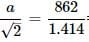= 609.6 pm

Test: Packing Efficiency (NCERT) - Question 3

### The edge length of sodium chloride unit cell is 564 pm. If the size of Cl- ion is 181 pm. The size of Na+ ion will be

Detailed Solution for Test: Packing Efficiency (NCERT) - Question 3

2(r+ + r-) = a
2(2Na+ + rCl-) = 564
2Na = 564/2 - 181 = 101 pm

Test: Packing Efficiency (NCERT) - Question 4

If the distance between Na+ and Cl- ions in NaCl crystals is 265 pm, then edge length of the unit cell will be?

Detailed Solution for Test: Packing Efficiency (NCERT) - Question 4

In NaCl crystal , Edge length = 2 x distance between Na+ and Cl
=2 x 265 = 530 pm

Test: Packing Efficiency (NCERT) - Question 5

The radius of Na+ is 95pm and that of Cl- is 181 pm. The edge length of unit cell in NaCl would be (pm).

Detailed Solution for Test: Packing Efficiency (NCERT) - Question 5

a = (r+ + r-) = 2(95 + 181) = 552 pm

Test: Packing Efficiency (NCERT) - Question 6

Copper crystallises in fcc with a unit cell length of 361 pm. What is the radius of copper atom?157 pm

Detailed Solution for Test: Packing Efficiency (NCERT) - Question 6

For fcc,
r = √2 / 4 x a = √2 / 4 x 361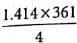= 127 pm

Test: Packing Efficiency (NCERT) - Question 7

Total volume of atoms present in a fcc unit cell of a metal with radius r is

Detailed Solution for Test: Packing Efficiency (NCERT) - Question 7

a = 2√2 r
Volume of the cell = a3 = (2√2 r)3 =  16√2r3
No. of shperes in fcc = 8 x 1/8 + 6 x 1/2 = 4
Volume of the four spheres = 4 x 4/3πr3
= 16πr3

Test: Packing Efficiency (NCERT) - Question 8

The relation between atomic radius and edge length 'a' of a body centred cubic unit cell:

Detailed Solution for Test: Packing Efficiency (NCERT) - Question 8

Distance between nearest neighbours, d = AD/2
In right angled △ABC,AC= AB2 + BC2
AC2 = a2 + a2  or AC = √2a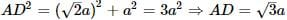∴ d = √3a / 2
Radius , r = d/2 = √3/4 a

Test: Packing Efficiency (NCERT) - Question 9

Edge length of unit cell of Chromium metal is 287 pm with the arrangement. The atomic radius is the order of:

Detailed Solution for Test: Packing Efficiency (NCERT) - Question 9

In bcc lattice, r = √3/4
r =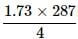= 124.27 pm

Test: Packing Efficiency (NCERT) - Question 10

The fraction of total volume occupied by the atoms present in a simple cube is

Detailed Solution for Test: Packing Efficiency (NCERT) - Question 10

For simple cube,
Radius(r) = a / 2 [a = edge length]
Volume of the atom =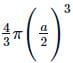Packing fraction =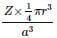Volume of the sphere (atoms) in an unit cell.
For simple cubic, Z = 1 atom
Packing fraction =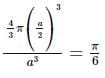## Chemistry Class 12

154 videos|396 docs|261 tests
Information about Test: Packing Efficiency (NCERT) Page
In this test you can find the Exam questions for Test: Packing Efficiency (NCERT) solved & explained in the simplest way possible. Besides giving Questions and answers for Test: Packing Efficiency (NCERT), EduRev gives you an ample number of Online tests for practice

## Chemistry Class 12

154 videos|396 docs|261 tests

### How to Prepare for NEET

Read our guide to prepare for NEET which is created by Toppers & the best Teachers(Scan QR code)